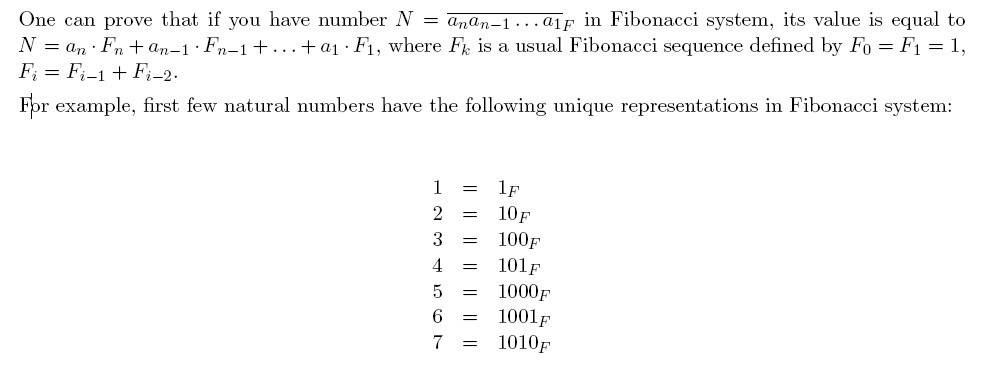# 2717. Fibonacci System

Little John studies numeral systems. After learning all about fixed-base systems, he became interested in more unusual cases. Among those cases he found a Fibonacci system, which represents all natural numbers in an unique way using only two digits: zero and one. But unlike usual binary scale of notation, in the Fibonacci system you are not allowed to place two 1s in adjacent positions.John wrote a very long string (consider it infinite) consisting of consecutive representations of natural numbers in Fibonacci system. For example, the first few digits of this string are 110100101100010011010. . .

He is very interested, how many times the digit 1 occurs in the N-th prefix of the string. Remember that the N-th prefix of the string is just a string consisting of its first N characters.

Write a program which determines how many times the digit 1 occurs in N-th prefix of John’s string.

### 输入格式

The input file contains a single integer N (0<N<10^15).

### 输出格式

Output a single integer ― the number of 1s in N-th prefix of John’s string.

### 样例

Input
21

Output
10


1 人解决，8 人已尝试。

1 份提交通过，共有 31 份提交。

9.9 EMB 奖励。